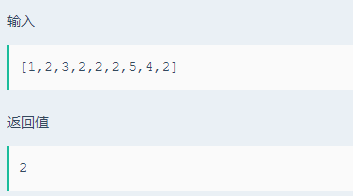## 【剑指offer简单部分9】数组中出现次数超过一半的数字（java）

2020/12/28 20:14:39 文章标签:

## 题目描述## 分析

``````import java.util.Arrays;
public class Solution {
public int MoreThanHalfNum_Solution(int [] array) {
Arrays.sort(array);
int num = array.length / 2;
for(int i = 0 ; i < array.length ; i++){
int count = 0;
for(int j = i ; j < array.length ; j++){
if(array[i] == array[j]){
count++;
if(count > num){
return array[j];
}
}
}
}
return 0;
}
}
````````````import java.util.Map;
import java.util.HashMap;
public class Solution {
public int MoreThanHalfNum_Solution(int [] array) {
Map<Integer, Integer> map = new HashMap<>();
//1、将数组放入HashMap中
for(int key : array){
map.put(key, map.getOrDefault(key,0) + 1 );//map.getOrDefault方法：当Map集合中有这个key时，就使用这个key值，如果没有就使用默认值defaultValue
}
//2、从HashMap中获取key，判断其出现次数是否超过数组长度的一半
//map.keySet()为获取key集，也就是数字
for(int key : map.keySet()){
//获取map.get(key)对应的value值，即数字重复出现的次数
if(map.get(key) > array.length / 2){
return key;
}
}
return 0;
}
}
``````暂无相关的数据...